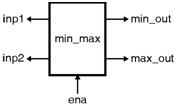Home > VHDL > Advanced VHDL > Procedure
Procedure : PROCEDURE is same as FUNCTION and has the same basic purposes. Further, a procedure returns more than one value. The two parts of PROCEDURE are 1) procedure body and 2) procedure call. PROCEDURE may have any number of IN, OUT, or INOUT parameters, which can be SIGNALS, VARIABLES, or CONSTANTS. Here, WAIT, SIGNAL declarations, and COMPONENTS are not synthesizable when used in a FUNCTION. Procedure Body : PROCEDURE procedure_test [parameter list] IS
```PROCEDURE procedure_test [parameter list] IS
[declarations]
BEGIN
(sequential statements)
END procedure_test;
```
In the syntax above,
```
specifies the procedure's input and output parameters; that is:

= [CONSTANT] constant_name: mode type;

= SIGNAL signal_name: mode type; or

= VARIABLE variable_name: mode type;
```

## Procedure Call :

Contrary to a FUNCTION, which is called as part of an expression, a PROCEDURE call is a statement on its own. It can appear by itself or associated to a statement (either concurrent or sequential). Examples of procedure calls :

```compute_min_max(in1, in2, 1n3, out1, out2);
-- statement by itself
divide(dividend, divisor, quotient, remainder);
-- statement by itself
IF (a>b) THEN compute_min_max(in1, in2, 1n3, out1, out2);
-- procedure call associated to another statement
```

## Procedure Location :

The typical locations of a PROCEDURE are the same as those of a FUNCTION (see Fig. 3.4.1). Again, though it is usually placed in a PACKAGE (for code partitioning, code reuse, and code sharing purposes), it can also be located in the main code (either in the ENTITY or in the declarative part of the ARCHITECTURE). PROCEDURE Located in the Main Code :

Figure shows the entity of min_max code which uses PROCEDURE. The PROCEDURE is located in the declarative part of the ARCHITECTURE.```LIBRARY ieee;
USE ieee.std_logic_1164.all;
ENTITY min_max IS
GENERIC (limit : INTEGER := 255);
PORT ( ena: IN BIT;
inp1, inp2: IN INTEGER RANGE 0 TO limit;
min_out, max_out: OUT INTEGER RANGE 0 TO limit);
END min_max;
ARCHITECTURE arch OF min_max IS
PROCEDURE sort (SIGNAL in1, in2: IN INTEGER RANGE 0 TO limit;
SIGNAL min, max: OUT INTEGER RANGE 0 TO limit) IS
BEGIN
IF (in1 > in2) THEN
max <= in1;
min <= in2;
ELSE
max <= in2;
min <= in1;
END IF;
END sort;
BEGIN
PROCESS (ena)
BEGIN
IF (ena='1') THEN sort (inp1, inp2, min_out, max_out);
END IF;
END PROCESS;
END arch;
```

PROCEDURE located in A PACKAGE :

This example is similar to above figure with the only difference is now the PROCEDURE is placed in a PACKAGE. Therefore, the PROCEDURE is reused and shared with other designs.

```LIBRARY ieee;
USE ieee.std_logic_1164.all;
PACKAGE pack IS
CONSTANT limit: INTEGER := 255;
PROCEDURE sort (SIGNAL in1, in2: IN INTEGER RANGE 0 TO limit;
SIGNAL min, max: OUT INTEGER RANGE 0 TO limit);
END pack;
PACKAGE BODY pack IS
PROCEDURE sort (SIGNAL in1, in2: IN INTEGER RANGE 0 TO limit;
SIGNAL min, max: OUT INTEGER RANGE 0 TO limit) IS
BEGIN
IF (in1 > in2) THEN
max <= in1;
min <= in2;
ELSE
max <= in2;
min <= in1;
END IF;
END sort;
END pack;
LIBRARY ieee;
USE ieee.std_logic_1164.all;
USE work.pack.all;
ENTITY min_max IS
GENERIC (limit: INTEGER := 255);
PORT ( ena: IN BIT;
inp1, inp2: IN INTEGER RANGE 0 TO limit;
min_out, max_out: OUT INTEGER RANGE 0 TO limit);
END min_max;
ARCHITECTURE arch OF min_max IS
BEGIN
PROCESS (ena)
BEGIN
IF (ena='1') THEN sort (inp1, inp2, min_out, max_out);
END IF;
END PROCESS;
END arch;
```